# Decoding malware via simple statistical analysis

Intro

Analyzing malware often requires code reverse engineering which can scare people away from malware analysis.

Executables are often encoded to avoid detection. For example, many malicious Word documents have an embedded executable payload that is base64 encoded (or some other encoding). To understand the encoding, and be able to decode the payload for further analysis, reversing of the macro code is often performed.

But code reversing is not the only possible solution. Here we will describe a statistical analysis method that can be applied to certain malware families, such as the Hancitor malicious documents. We will present this method step by step.

Examples

First we start with a Windows executable (PE file) that is BASE64 encoded. In BASE64 encoding, 64 different characters are used to encode bytes. 64 is 6 bits, hence there is an overhead when encoding in BASE64, as encoding one byte (8 bits) will require 2 BASE64 characters (6 bits + 2 bits).

With byte-stats.py, we can generate statistics for the different byte values found in a file. When we use this to analyze our BASE64 encoded executable, we get this output:In the screenshot above see that we have 64 different byte values, and that 100% of the byte values are BASE64 characters. This is a strong indication that the data in file base64.txt is indeed BASE64 encoded.

Using the option -r of byte-stats.py, we are presented with an overview of the ranges of byte values found in the file:The identified ranges /0123456789, ABCDEFGHIJKLMNOPQRSTUVWXYZ and abcdefghijklmnopqrstuvwxyz (and single charcter +) confirm that this is indeed BASE64 data. Padded BASE64 data would include one or two padding characters at the end (the padding character is =).

Decoding this file with base64dump.py (a BASE64 decoding tool), confirms that it is a PE file (cfr. MZ header) that is BASE64 encoded.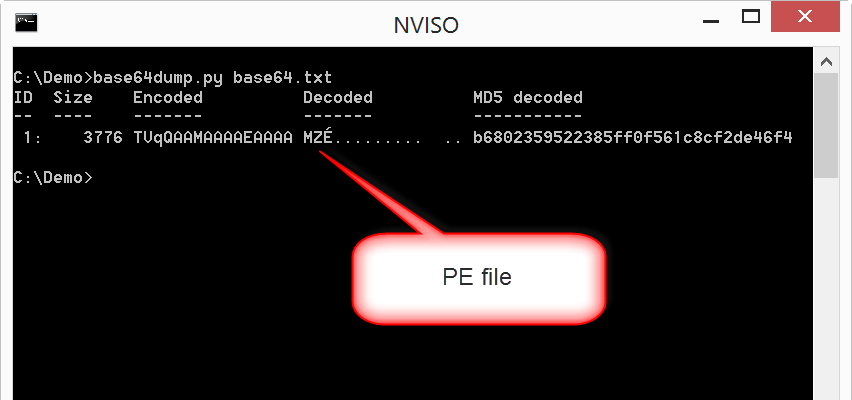Now, sometimes the encoding is a bit more complex than just BASE64 encoding.

Let’s take a look at another sample: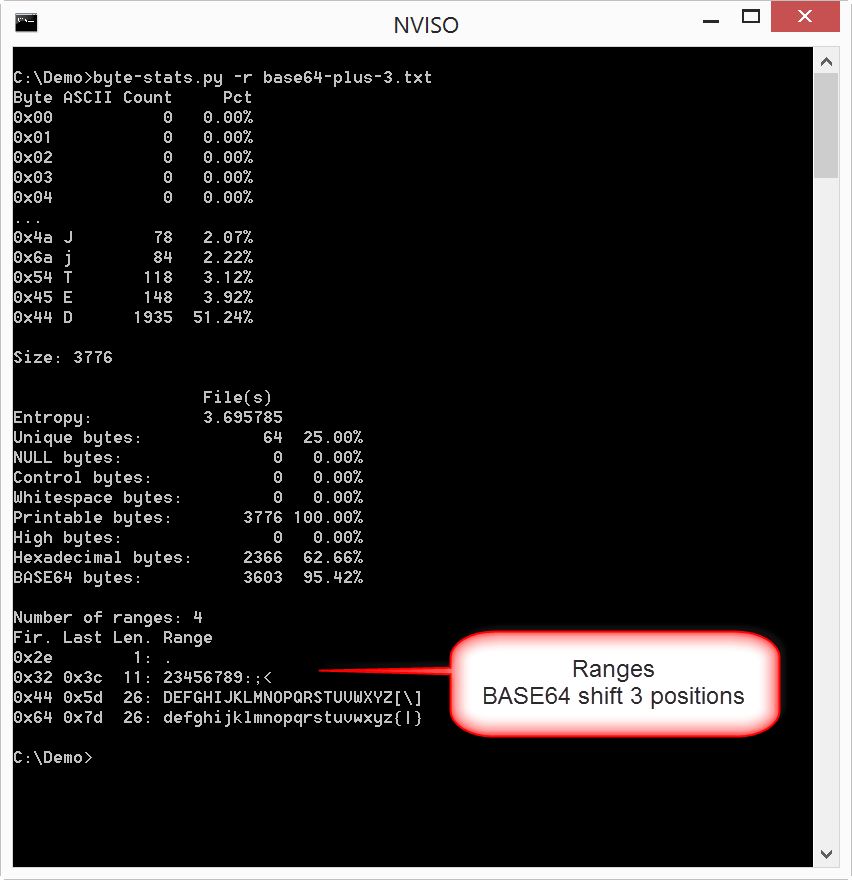The range of lowercase letters, for example, starts with d (in stead of a) and ends with } (in stead of z). We observer a similar change for the other ranges.

It looks like all BASE64 characters have been shifted 3 positions to the right.

We can test this hypothesis by subtracting 3 from every byte value (that’s shifting 3 positions to the left) and analyzing the result. To subtract 3 from every byte, we use program translate.py. translate.py takes a file as input and an arithmetic operation: operation “byte – 3” will subtract 3 from every byte value.

This is the result we get when we perform a statistical analysis of the byte values shifted 3 positions to the left: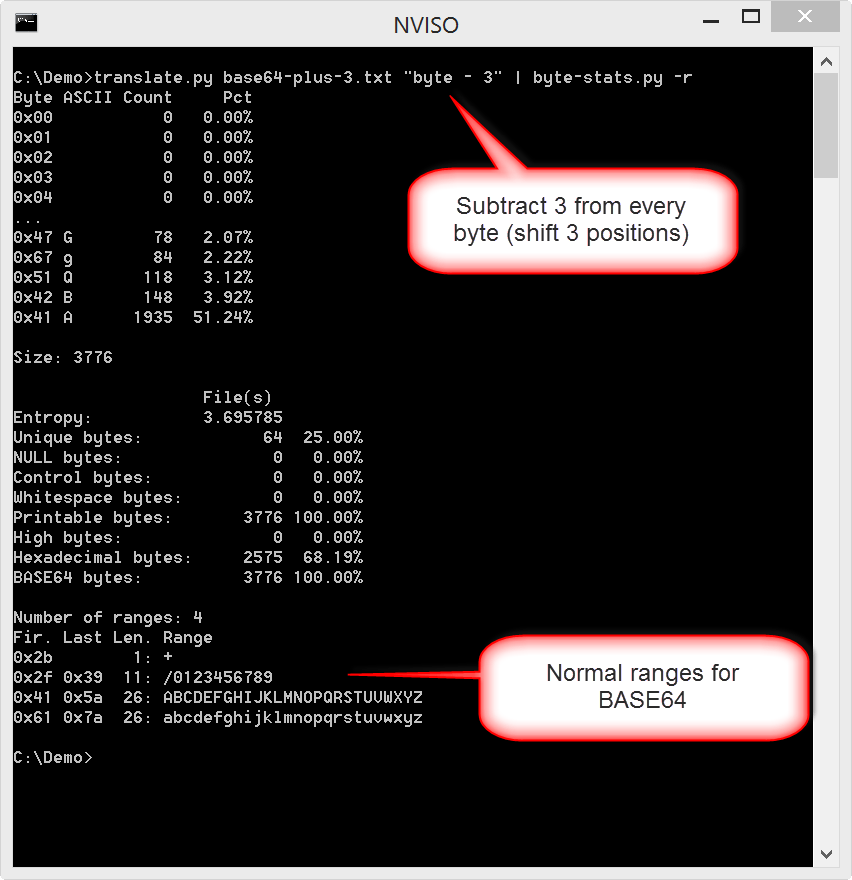In the screenshot above we see 64 unique bytes and all bytes are BASE64 characters. When we try to decode this with base64dump, we can indeed recover the executable:Let’s move on to another example. Malicious documents that deliver Hancitor malware use an encoding that is a bit more complex: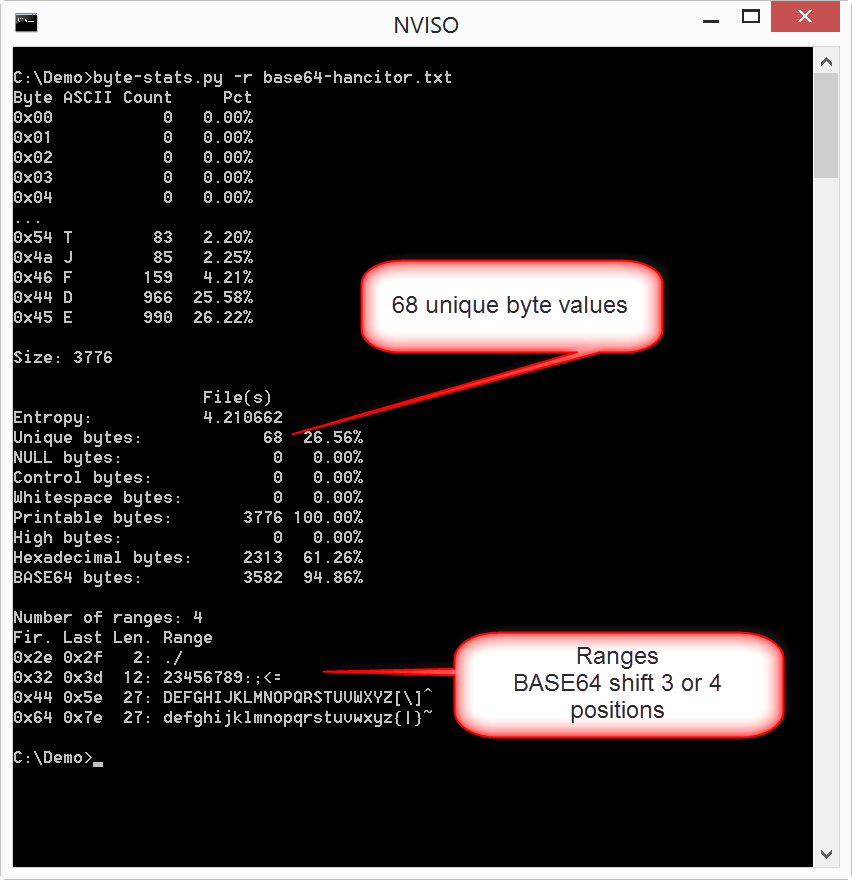This time, we have 68 unique byte values, and the ranges are shifted by 3 positions when we look at the left of a range, but they appear to be shifted by 4 positions when we look at the right of a range.

How can this be explained?

One hypothesis, is that the malware is encoded by shifting some of the bytes with 3 positions, and the other bytes with 4 positions. A simple method is to alternate this shift: the first byte is shifted by 3 positions, the second by 4 positions, the third again by 3 positions, the fourth by 4 positions, and so on …

Let’s try out this hypothesis, by using translate.py to shift by 3 or 4 positions depending on the position: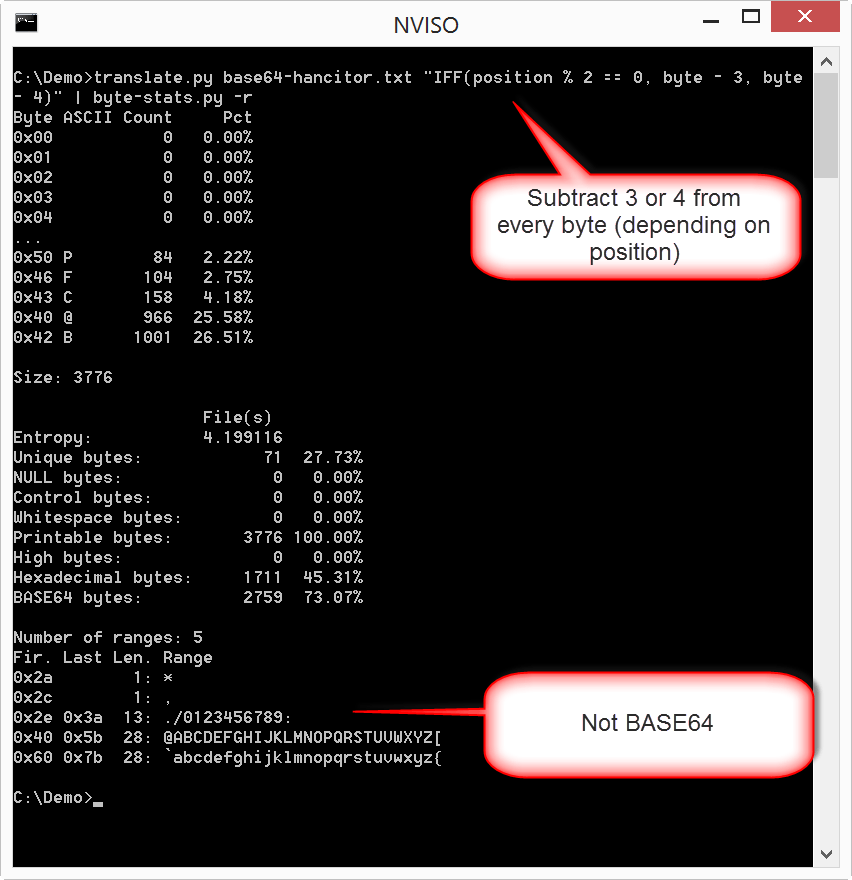Variable position is an integer that gives the position of the byte (starts with 0), and position % 2 is the remainder of dividing position by 2. Expression position % 2 == 0 is True for even positions, and False for uneven positions. IFF is the IF Function: if argument 1 is true, it returns argument 2, otherwise it returns argument 3. This is how we can shift our input alternating with 3 and 4.

But as you can see, the result is certainly not BASE64, so our hypothesis is wrong.

Let’s try with shifting by 4 and 3 (instead of 3 and 4):This time we get the ranges for BASE64.

Testing with base64dump.py confirms our hypothesis:Conclusion

Malware authors use encoding schemes that can be reverse engineered by statistical analysis and testing simple hypotheses. Sometimes a bit of trial and error is needed, but these encoding schemes can be simple enough to decode without having to perform reverse engineering of code.